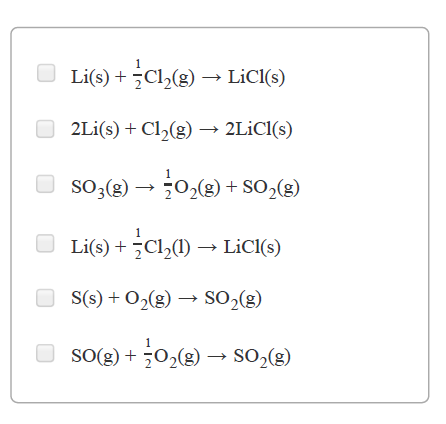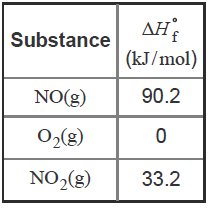# Problem: Part A. For which of the following reactions is ΔH°rxn equal to ΔH°f of the product(s)? You do not need to look up any values to answer this question.Check all that apply.The standard heat of formation, ΔH∘f , is defined as the enthalpy change for the formation of one mole of substance from its constituent elements in their standard states. Thus, elements in their standard states have ΔH°f = 0. Heat of formation values can be used to calculate the enthalpy change of any reaction.Consider, for example, the reaction2 NO(g) + O2(g) ⇌ 2 NO2(g)with heat of formation values given by the following table:∆Hrxn = ∆H°fproducts-∆H°freactants∆Hrxn = 2 (33.2) - 290.2+0∆Hrxn = -114 kJ

###### FREE Expert SolutionView Complete Written Solution
###### Problem Details

Part A. For which of the following reactions is ΔH°rxn equal to ΔH°f of the product(s)?

You do not need to look up any values to answer this question.

Check all that apply.The standard heat of formation, ΔHf , is defined as the enthalpy change for the formation of one mole of substance from its constituent elements in their standard states. Thus, elements in their standard states have ΔH°f = 0. Heat of formation values can be used to calculate the enthalpy change of any reaction.

Consider, for example, the reaction

2 NO(g) + O2(g) ⇌ 2 NO2(g)

with heat of formation values given by the following table: